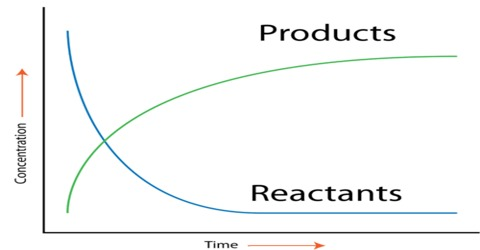Chemistry

# Experimental Determination of the Rate of a ReactionExperimental Determination of the Rate of a Reaction

Various experimental procedures have been used to determine the rates of reactions. For different reactions different methods may have to be used. As we have seen, for the determination of the rate of a reaction one has to determine the concentration of the reactant or the product at different intervals of time. A few examples are mentioned.

(a) Hydrogen peroxide decomposes in the presence of manganese (IV) oxide according to the equation below:

2H2O2 (l) → H2O (l) + O2 (g)

A gas is evolved as a result of the reaction. So the rate may be found by measuring the volume of gas evolved at different times.

(b) If a reaction is slow that a fixed volume of sample of the reaction mixture can be withdrawn at intervals and the concentration determined by chemical analysis. As the reaction continues to proceed, in the sample taken it is the practice to “freeze” the reaction before analysis. Freezing means to substantially forum the raw of the reaction. This can be done

• by rapidly cooling the sample to a low temperature
• by adding a chemical which will remove one of the reactants without erecting the others or
• by dilution in a large volume

An example is the hydrolysis of ethyl acetate by aqueous sodium hydroxide solution.

CH3-COOC2H5 (aq) + NaOH (aq) → CH3COONa (aq) + C2H5OH (aq)

The reaction can be followed by titration of the samples with standard hydrochloric acid solution as the concentration of NaOH gradually decreases as the reaction proceeds.